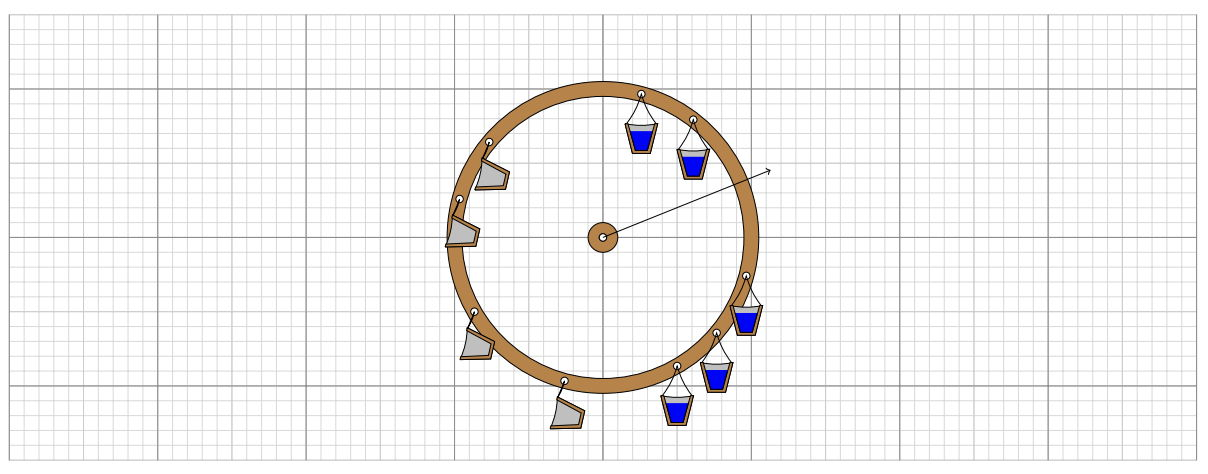시간 제한 메모리 제한 제출 정답 맞은 사람 정답 비율
2 초 512 MB 0 0 0 0.000%

문제

A water wheel is a device for converting the potential energy of water into the kinetic energy of rotation. We have attached buckets along our otherwise perfectly-balanced wheel. Each bucket has a specific weight when empty, and a specific weight when full of water, both in kilograms.

Whenever a bucket reaches the top of the water wheel, it is filled to its maximum capacity. When the bucket reaches the bottom, diametrically opposite, it is emptied again.Figure D.1: Illustration of Sample Input 1 at approximately 15.0 degrees clockwise from the start. The centre of mass is drawn as a vector from the origin.

The acceleration of the wheel depends on the horizontal distance between the centre of mass of the wheel and its axis of rotation. Centre of mass of the wheel at some time is defined as the sum of the co-ordinates of the buckets at that time multiplied by their weight.

Our water wheel has a radius of exactly 1 metre. What is the maximum positive x-component of centre of mass achieved by the wheel at any angle?

입력

• The first line of input contains the number of buckets on the wheel, n (2 ≤ n ≤ 105).
• The remaining n lines of input each contain a description of a bucket, in angular order:
• the decimal clockwise angle of the ith bucket in degrees, di (0.0 ≤ d ≤ 360.0),
• the decimal weights of this bucket when empty and full, ei and fi (0 ≤ ei ≤ fi ≤ 104).

출력

Output the maximum horizontal component of the centre of mass of the wheel over all possible angles. Your answer should be within an absolute or relative error of at most 10−6.

9
0.000 0.5 1.8
22.50 1.0 1.7
90.00 0.5 1.0
115.0 1.0 1.2
135.0 1.0 1.2
180.0 1.0 1.2
225.0 1.0 1.2
270.0 1.0 1.2
295.0 1.0 1.2

예제 출력 1

1.65664252966349700991